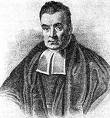## Friday, December 08, 2006

### Reverend Bayes and God

An interesting article in ScientificAmerican.com discusses What is Bayes's theorem, and how can it be used to assign probabilities to questions such as the existence of God?

The article describes Bayes' theorem which relates the conditional of event A given B with the event B give A. This is a very powerful theorem that has many practical applications. The reason is that we usually want to predict an event in the future (A) given an event in the past (B), but that is hard to do. Instead, we try the opposite scenario: if event A happens in the future, what is the probability that it was preceded by event B?

The pwerful Naive Bayes classifier is based on Bayes' theorem, with a slight modification.

The final part of the article discusses three books that have tried to use Bayes' theorem to compute the probability that God exists. The author very reasonably argues that this is not the right tool for answering this question.An interesting question is whether Reverend Thomas Bayes himself reached his theorem while contemplating on theological issues? Did he even try to marry his theology and science?